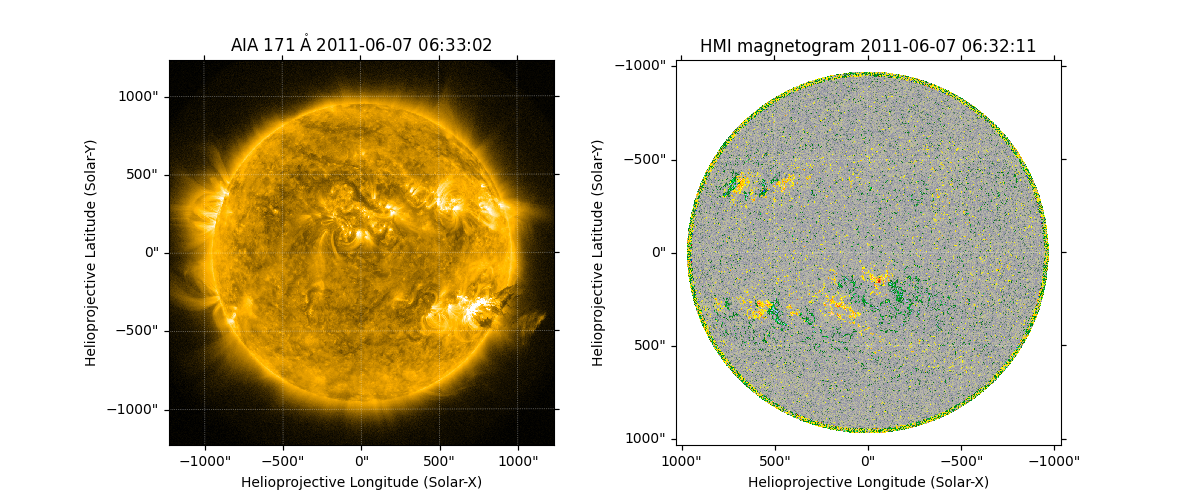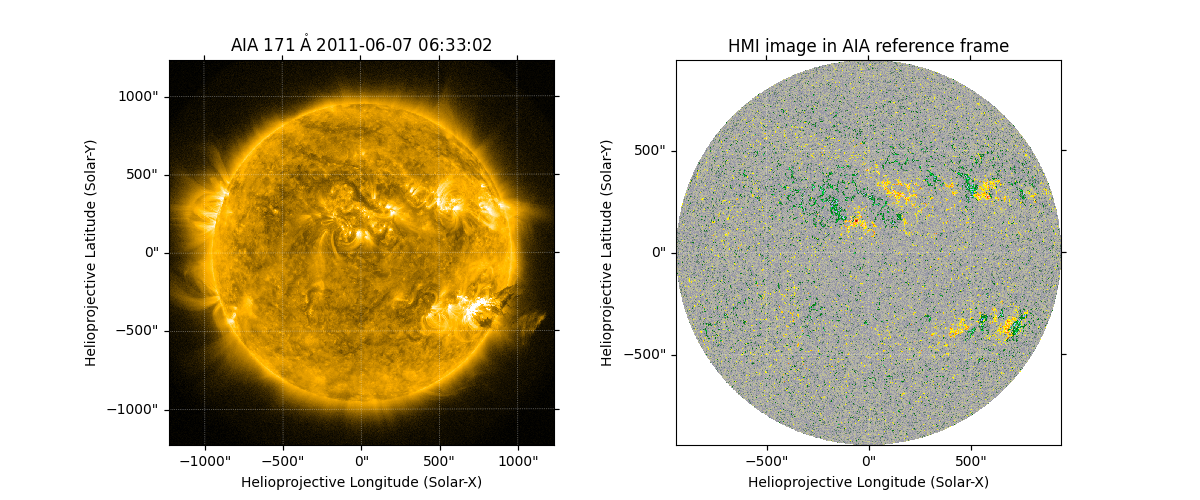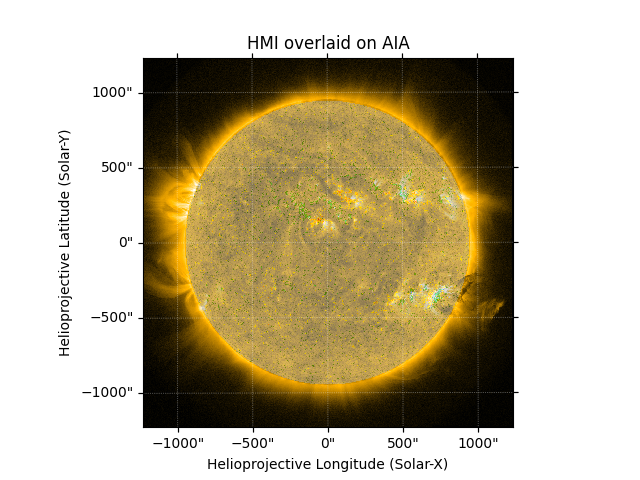# Auto-Aligning AIA and HMI Data During Plotting#

This example shows how to auto-align two images with different reference frames during plotting.

Here we use the optional keyword autoalign when calling Map’s plot() method. The reference frames are defined by the respective World Coordinate System (WCS) information.

See Aligning AIA and HMI Data with Reproject for an alternate approach to image alignment, where one of the maps is modified prior to plotting, and thus is available for purposes other than plotting.

import matplotlib.pyplot as plt

import astropy.units as u

import sunpy.data.sample
import sunpy.map


We use the AIA image and HMI image from the sample data. For the HMI map, we use the special HMI color map, which expects the plotted range to be -1500 to 1500.

map_aia = sunpy.map.Map(sunpy.data.sample.AIA_171_IMAGE)
map_hmi = sunpy.map.Map(sunpy.data.sample.HMI_LOS_IMAGE)
map_hmi.plot_settings['cmap'] = "hmimag"
map_hmi.plot_settings['norm'] = plt.Normalize(-1500, 1500)


Plot both images side by side. Note that the HMI image is oriented “upside down” relative to the AIA image.

fig = plt.figure(figsize=(12, 5))
map_aia.plot(axes=ax1, clip_interval=(1, 99.9)*u.percent)
map_hmi.plot(axes=ax2)<matplotlib.image.AxesImage object at 0x7f2dfea1a3e0>


Setting autoalign=True allows plotting the HMI image onto axes defined by the AIA reference frame. In contrast to the above code block, we intentionally set the projection for the axes to be the AIA map instead of the HMI map. We also need to manually set the plot limits because Matplotlib gets confused by the off-disk parts of the image. The HMI image now has the same orientation as the AIA image.

Note that off-disk HMI data are not retained by default because an additional assumption is required to define the location of the HMI emission in 3D space. We can use assume_spherical_screen() to retain the off-disk HMI data. See Reprojecting Using a Spherical Screen for more reference.

fig = plt.figure(figsize=(12, 5))
map_aia.plot(axes=ax1, clip_interval=(1, 99.9)*u.percent)
map_hmi.plot(axes=ax2, autoalign=True, title='HMI image in AIA reference frame')
ax2.axis(ax1.axis())(-0.5, 1023.5, -0.5, 1023.5)


We can directly plot them over one another, by setting the transparency of the HMI plot.

fig = plt.figure()